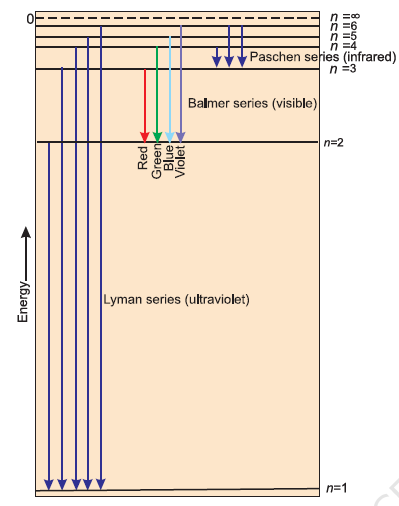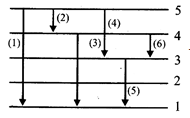# Line Spectrum of Hydrogen

## Line Spectrum of Hydrogen

When hydrogen gas present on discharge tube passed through high voltage & low pressure, the radiations emitted passed through spectroscope and spectrum is obtained on photographic plate. Hydrogen spectrum contains several lines known as series. The series present in hydrogen spectrum are:-
 Series n1 n2 Spectral region Lyman 1 2,3,4,.. Ultraviolet Balmer 2 3,4,5…. Visible Paschen 3 4,5,6,7.. Infrared Brackett 4 5,6,7… Infrared Pfund 5 6,7,8… Infrared

Balmer showed that wave no. of spectral lines in visible region is given by
$= 109677( \frac {1}{2^2} - \frac {1}{n^2})$  cm-1
where n is an integer equal to or greater than 3 (i.e., n = 3,4,5,....)
Wave no. for any two series is obtained by
$= R( \frac {1}{n_1^2} - \frac {1}{n_2^2})$   cm-1
n1, n2 = level of series
The value R = 109,677 cm-1 is called the Rydberg constant for hydrogen.
The below shows the Lyman, Balmer and Paschen series of transitions for hydrogen atomQuestion 1
Calculate the wavelength of the spectral line in the spectrum of hydrogen tom when n = 3 in Balmer formula.
Solution
$\frac {1}{\lambda } = R (\frac {1}{(n_1^2}- \frac {1}{n_2^2 })$
For Balmer series n1=2
$\frac {1}{\lambda } = R (\frac {1}{(2^2}- \frac {1}{n_2^2 })$
Now for n1=3
$\frac {1}{\lambda } = R (\frac {1}{(2^2}- \frac {1}{3^2 })$
λ= 36/5 R = 6.792 × 10-5 cm

Question 2
In hydrogen atom the electron jumps from 3rd orbit to 1st orbit. Find out fequency & wavelenght of spectrum line.
Solution
$\frac {1}{\lambda } = R (\frac {1}{(n_1^2}- \frac {1}{n_2^2 })$
$\frac {1}{\lambda } = R (\frac {1}{(1^2}- \frac {1}{3^2 })$
$\frac {1}{\lambda }=109677 (1- \frac {1}{9})$
λ= = 9/8 × 1/(1.09 × 105 )
= 1.02 × 10-5 cm.= 102 × 10-5 m
Now, C = νλ

ν=(3 × 108)/(102 × 10-5 )
=(3 × 1013)/102
= 2.67 × 1011 s-1

Question 3
What is the wavelength of light emitted when the electron in a hydrogen atom undergoes transition from an energy level with n = 4 to an energy level with n = 2?
Solution
λ= ?
n = 4, n = 2
$\frac {1}{\lambda } = R (\frac {1}{(n_1^2}- \frac {1}{n_2^2 })$
$\frac {1}{\lambda } = R (\frac {1}{(2^2}- \frac {1}{4^2 })$
? = 16 / 109678 × 3 cm = 486 nm
Question 4
Calculate the wave number for the longest wavelength transition in the Balmer series of atomic hydrogen.
Solution
$\frac {1}{\lambda } = R (\frac {1}{(n_1^2}- \frac {1}{n_2^2 })$
For Balmer series n1=2
$\bar{\nu}=\frac {1}{\lambda } = R (\frac {1}{(2^2}- \frac {1}{n_2^2 })$
To get maximum wavelength, wave no. must be least. For wave no. to be least n2 should be minimum
Therefore n2 = 3
$\bar{\nu} = R (\frac {1}{(2^2}- \frac {1}{n_2^2 })$
$\bar{\nu} = 1.09 \times 10^5 \times 5/36 = 1.5 \times 10^4$ cm-1

Question 5
Which of the following is true
(a) Lyman series is a continuous spectrum
(b) Paschen series is a line spectrum in the infrared
(c) Balmer series is a line spectrum in the ultraviolet
(d) The spectral series formula can be derived from the Rutherford model of the hydrogen atom
Solution

Question 6
In a collection of H-atoms, all the electrons jump from n = 5 to ground level finally (directly or indirectly), without emitting any line in Balmer series. The number of possible different radiations is:
(a) 10
(b) 8
(c) 7
(d) 6
Solution
(d)